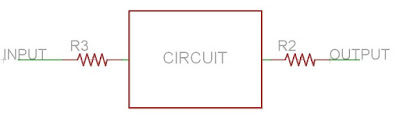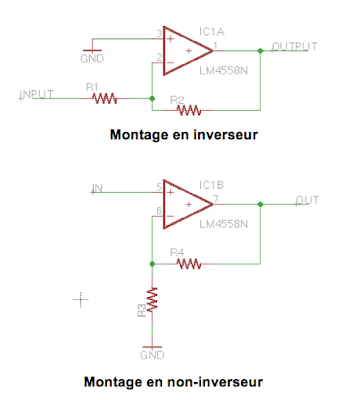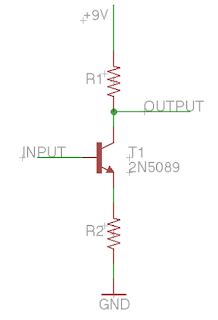# All you need to know about resistors in guitar pedals

In this article (that will be part of a series of articles about the different components you can find in a guitar pedal), we will try to decipher the role of a component you surely already know: the resistor! The resistor is a simple 2-legged component. It is the basic brick of every electronic circuit, like the simplest cubic Lego brick. You will find resistors everywhere, it has many, many, many possible uses. Here, we will be interested in its role in guitar pedals.

## What does it looks like?

The common resistor looks like a brown / blue cylinder, with rings of different colours and 2 legs.Everything is about color with resistors (being color blind is quite painful with it... If you are, there is an app to help you). The overall color of the resistor indicates its composition: carbon comp are dark-brown, carbon film are beige and metal film resistor are blue / cyan like the picture above. Which ones are the best for guitar pedals? Check my post: how to choose resistors for guitar pedals.

The coloured rings allow you to know what is the value of the resistor, written in Ohms. Each colour correponds to a number:Here for instance, we can read on the 3 first rings: "1", "5" and "0" = 150
The 4th number is 100, so we multiply the first value by 100 : 150 x 100 = 15000 = 15k

The last ring indicates the tolerance of the component. Tolerance is the possible maximal difference between the theoritical value indicated on the component (15k for instance), and its real value (14,99k for example). Practicaly speaking, metal film resistors that are used most of the times in guitar pedals have a 1% tolerance value (that is precise enough!), but some "precision" resistor can have tolerances down to 0.01%! They are often use in measurement devices like multimeters. In our case, 1% is really a sufficient value, there will be no audible difference if you swap a 100 ohms resistor by a 99 ohms one...

Knowing this code is not essential, you learn it gradually by practicing! If you forget, Google is here for you anyway ;)Inside the resistor, below this coloured capsule, there is a resistive film arranged as a spiral, composed of metal or carbon (carbon film or metal film!). The longer this film is, the higher the value of the resistor will be.Here for instance, the upper resistor is 27 ohms, the middle one 330 ohms and the bottom one 3.3 Mega Ohm (3 300 000 Ohms !)

The resistor has 2 symbols:On the left is the european symbol (R5), and on the right is the american symbol (R6). It is not the only component with two different symbols. Capacitors also have different symbols between Europe and America. For resistors, I actually prefer the american symbol that evoques maybe more the role played by the resistor and the resistive strip. It is also more common to find it on guitar effects schematics.

If you want to buy cheap (but nice quality) resistors, I have this great deal.

## What is its role?

A resistor, as its name suggests, resits against the flow of current. It will "absorb" a bit a current and transform it into heat. Thus, current will diminish when going through a resistor. This will diminish the voltage of the signal. The tension of the resistor is characterized this by the relationship:

U=R x I
with U = tension of the resistor (Volts), R = resistance (ohms)
and I= current intensity (amperes)

So basically, a part of the guitar signal will be absorbed to generate this tension. Thus, we can modulate the signal amplitude by modifying the resistor's value. This is really useful in a lot cases. Indeed, yout guitar signal is an alternative tension! If you want to learn more about it, read my article about electric guitar signal.

When the signal goes through a resistor, its amplitude decreases. A lower amplitude signal is simply a lower volume signal!With a resistor, it is thus possible to reduce guitar volume! However, a resistor can only diminish the voltage amplitude. To amplify a signal, you need semiconductor devices like transistors or an OP amp.
The resistor has a lot of other applications of course! Lets see of few of them together.

## How are resistors used in guitar pedals?

A resistor can have many, many applications... Here are some resistors standard applications that you can find in guitar pedals.

### 1. Adjusting the input or output level of an effect

Here is an example from the Big Muff circuit. An input resistor adjusts the amplitude of the input signal that will be amplified by a transistor. By changing this resistor, you can change the amount of signal entering the circuit, and thus you can change the overall gain of the pedal. Inversely, if its value is increased, the signal will be less amplified and you will have a smoother, less gainy Big Muff!You can do the same thing at the end of the circuit to set the output volume! Usually, we use a potentiometer wired as a variable resistor, so you can adjust the final volume.

### 2. Pulldown resistors

When a circuit is off, some voltage can stay at the beginning the disconnected input of the circuit or in capacitors. When the pedal is turned on, the voltage goes through the circuit and causes these annoying "popping noises". To avoid that, we can add a pulldown resistor at the entry or / and output of the circuit:Generally, a resistor with a high value (like 1M) is connected to the ground on the signal path. Thus, the excess of current that goes through the circuit when the pedal is turned on is absorbed by this resistor, and you avoid these loud noises.

### 3. Adjusting the gain of a transistor or an Operational Amplifier

Generally, transistors or OP-amps are used in a really simple schematic that allows you to amplify the signal: common emitter / collector for the transistor, inverter or non-inverter for the OP-amp. Each of these simple schematics usually use resistors. These resistors will define the gain of the circuit.For an OP-amp, the gain value is easy to calculate: for an inverter circuit, it will be R2/R1 and for a non-inverter circuit, it will be 1 + R4/R3. Thus, you can adjust the gain in a very precise manner! It is used in the Rub-A-Dub reverb to adjust the entry and output level of the effect.For a transistor wired in common emitter like here, you can adjust the value of the resistor connected to the ground (bigger value : less gain), or to the +9V bus (bigger value: more gain). You can already use this tip in the Big Muff circuit!

### 4. Voltage divider

This really simple scheme allows you to adjust the voltage that you provide to some parts of the circuit.The delivered tension V1 is simply : 9V x R2 / (R1 + R2).
If you put the same value to R1 and R2, you simply divide your input tension (here, 9V) by two.
This can be really useful for some components. For instance, a lot of operational amplifiers (AOP) need a 4.5V tension to work properly. It can also be really useful to bias the base of a transistor. You can see such a scheme in the volume / output section of the Big Muff circuit.

A potentiometer is simply a variable resistor! Any resistor of a circuit can be replaced by a potentiometer, so that the user can modify manually a value. We will see how it works in detail in another article.

Just an example: if you put a potentiometer in the loop of an operational amplifier, you can make the gain vary just by turning the potentiometer! Ideal for a volume boost, or to set the maximal amount of gain with clipping diodes. You can find such thing in the Jan Ray / Timmy circuit.

You can also put a potentiometer wired as a variable resistor to the ground at the end of the circuit, just before the output, so you can set the final volume! It is the simplest possible volume control, which you can find in many circuits, including the Fuzz Face.

## An example

Lets see an example together. Here is a simple circuit, the Linear Power Booster 1 (LPB1), from Electro Harmonix. It is the first boost using a silicon transistor.As you can see, there are five resistors (6 if we include the boost potentiometer) in this circuit. With all the things we saw before, you should be able to determine what is each resistor's role in this circuit.

The smarter guys (girls?) among us should even know which resistors to change to have more or less gain with this boost!

Some help with reading the schematic: first, try to see what path the signal is going through. For that, link the input to the output of the circuit, and you can see where the signal is going. Here, it goes through C1, Q2, C2, and then the "Boost" potentiometer. Now, you should be able to find the role of the resistors.
If you do not, do not worry! Here is some help: http://www.coda-effects.com/2015/09/lpb1-mini-pcb.html

Pour aller plus loin :
Nice guide from guitarPCB, about all guitar fx components.
Pulldown resistors: AMZ FX blog

Previous
Next Post »
##### 9 CommentCan you do one of these on capacitors?What about swapping out a 15k for a 12k resitor? i need this for a klon centaur and the resisitors ive been sent are 4 times the size and wont fit on the board.. :( can i jsut use a slightly higher resitance resistor,. will it effect my circuit to much?No, not really... that little variance should be ok... try it and see if it sounds how you like it.I bought a bunch of 8k2 5% resistors.

They're grey with two red bands and one gold band, would these be able to work in a guitar pedal?